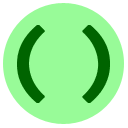# 4: Types

From this lesson onwards, examples and code input boxes have buttons labelled Open in console and Visualize. Use them to help debug and explore the code.

In the Hello, World! program we saw that Python was able to repeat a sentence back to us. We have also seen several examples of arithmetic with numbers. Numbers and sentences are fundamentally different objects, and it causes a Python error when you try to mix them in the wrong way:

Example
Trying to compare a string and a number.

As you can see, we get an error saying that the two arguments to `max` are of different types. The error is a good introduction to the rest of the lesson:

• `"Hello, World!"` is a string value, which is shown as `str` in Python. A string is any sequence of numbers, letters, and punctuation; we will learn more about them in lesson 7A.
• `35` is an integer value, which is shown as `int` in Python. An integer just means a whole number; for example, 42, -12, and 0 are integers.

Using an object of a bad type is a very common cause of errors in programs. It is like trying to drink a sandwich: you can't do it because you can only drink things of liquid type, and a sandwich is of solid type.

You can determine the type of an object by calling the `type` function on it.

Example
Some examples of types.

(The meaning of `class` is similar to `type`.) The above example demonstrates that numbers are further divided into two different types, `int` which we mentioned above, and `float`, which is used for storing decimal numbers. You should think of `float`s as inexact or approximate values (we will explain more in lesson 7B). You can usually mix `float` values with `int` values, and the result will be another `float`.

Example
Mixing an `int` and a `float`.

In fact, what Python really does when you mix a `float` with an `int` is that it converts the `int` to a `float`, and then works with the two `float`s.Multiple Choice Exercise: Floating
If we change `1.2` to `1.5` in the above program, what is first line of output?
Correct! We have to explain two facts here, to see why `z` was printed as `3.0`.
• Computing `x * y` is mixing an `int` and a `float`, which Python treats as two `float`s, giving back `z` as a `float`.
• The mathematical value of `z` is 1.5 times 2, which is 3, but stored in inexact decimal form, of type `float`. When Python prints any `float`, even if its value is a whole number, it is printed ending with `.0` to clarify that it is an inexact value.
Also, note that the value and type of `y` never changed.

It is often necessary to change data from one type to another type. Just as you can convert a sandwich from solid to liquid form by using a blender, you can change data from one type to another type using a typecast function. You write the name of the desired new type in the same way as a function call, for example

```x = float("3.4")
print(x-1)```
changes the string `"3.4"` to the float `3.4`, and then prints out `2.4`. Without the typecast, the program would crash, since it cannot subtract a number from a string.Sometimes, Python does let you combine strings and numbers using arithmetic operators. The statement `print("hots" * 2) `prints `hotshots`. Python's rule is that multiplying a string s by an integer n means to put n copies of the string one after another. We'll see later that "addition of two strings" is also well-defined in Python.

Various typecasts behave differently:

• converting a `float` to an `int` loses the information after the decimal point, e.g. `int(1.234)` gives `1`, and `int(-34.7)` gives `-34`.
• converting a `str` to an `int` causes an error if the string is not formatted exactly like an integer, e.g. `int("1.234")` causes an error.
• converting a `str` to a `float` causes an error if the string is not a number, e.g. `float("sandwich")` causes an error.

A common use of typecasting that we will see soon is to convert user input, which is always a string, to numerical form. Here is a quick illustration.

Example
Example of typecasting.

Here is one more exercise to finish the lesson.Because there are now lots of editor commands, some have been moved into the menu labelled More actions...Coding Exercise: Tasty Typecasting
Write a program to help you feed your friends at a party by doing some math about square pizzas. Assume the grader defines a string `inputStr` for you, which is a decimal string meaning the side length L of the pizza in cm. The area of the pizza should be computed using the formula A = L*L. Then, assuming that each person needs to eat 100 cm2 of pizza, compute the number of people it can feed, rounded down to the nearest integer. Hint

Example: if `inputStr` is `"17.5"`, the area will be 306.25 cm2, so `3` is the correct output.

Once you are done, head over to the next lesson.